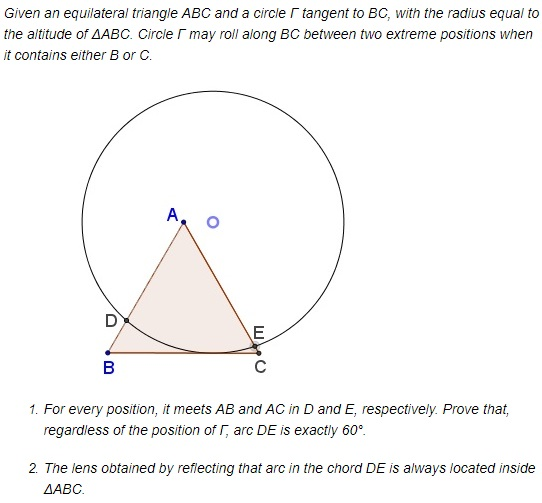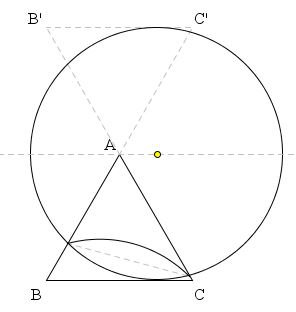# A Circle Rolling in an Equilateral Triangle What is this about? A Mathematical Droodle

ExplanationThe applet may suggest the following statement:### Proof

Let B' be the reflection of C in A and C' that of B. Let λ be the line through A parallel to BC. We see that ΔAB'C' is the symmetric image of ΔABC in λ and so are the two halves of circle Γ cut off by λ. It follows that the two arcs cut off by Γ inside the two triangles are equal.On the other hand, ∠BAC formed by two lines BC' and B'C could be looked at as formed by two intersecting chords. It therefore equals half the sum of the two arcs and, since they are equal, to either of them. But ∠BAC = 60° and so are the arcs, independent of the position of Γ.

At the extreme positions of Γ the angle formed by DE and BC is 30° and DE serves as a bisector of either ∠B or ∠C. In-between the angle is less than 30°. This angle is equal to the angle formed by DE with the upper border of the lens which then is also less than 30°. But this exactly means that the upper arc crosses neither AB nor AC.

Obviously, the lens fit snuggly in the equilateral triangle and may rotate there always touchingits sides. The lens is a simplified analog of shapes of constant width

### References

1. D. O. Shklyarsky, N. N. Chentsov, I. M. Yaglom, Selected Problems and Theorems from Elementary Mathematics, Geometry, 1952 (in Russian)• A problem with equilateral triangles: an interactive illustration
• Six Incircles in Equilateral Triangle
• Equilateral Triangle, Straight Line and Tangent Circles
• Equilateral Triangles on Diagonals of Antiequilic Quadrilateral
• Equilateral Triangles On Sides of a Parallelogram
• Equilateral Triangles On Sides of a Parallelogram II
• Equilateral Triangles on Sides of a Parallelogram III
• Triangle Classification
• Equilateral Triangles and Incircles in a Square
• Equal Areas in Equilateral Triangle
• Viviani's Theorem
• Regular Polygons in a Triangular Grid
• Common Centroids Lead to Equilateral Triangle
•